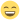## Effect size calculations

Discuss statistics related things

## Effect size calculations

Hi

I am a naive use of JAMOVI. I tried to calculate the effect size for a paired t-test using JAMOVI. I checked the cohen's d using MOTE package and these numbers are quite different. Below is the code that replicates my output:

library(jmv)
data('bugs', package = 'jmv')
jmv::ttestPS(bugs, pairs = list(list(i1 = 'LDLF', i2 = 'LDHF')), hypothesis = "twoGreater", meanDiff = TRUE, effectSize = TRUE, ci = TRUE, desc = TRUE, plots = FALSE)

OUTPUT SHOWS cohen's d= -0.697

library(MOTE)
d.dep.t.avg(m1 =5.72 , m2 = 7.38, sd1 = 2.71, sd2 = 2.52 , n = 91, a = .05)

OUTPUT SHOWS cohen's d= -0.6347992

This not so much a rounding errors. Kindly advise

Cheers

Posts: 2
Joined: Tue Aug 28, 2018 1:00 pm

## Re: Effect size calculations

the sd1 of 2.71 looks to be based on the full 93 observations of LDLF, however LDHF has two missing values, and so these two cases need to excluded from LDLF as well.

if i take the sd1 value of 2.67 from the t-test descriptives, i get the same results.

(for the record MOTE is a *super-rough* package at this stage - i had to roll back a few commits to find a version which worked. maybe pick a package that's at least on CRAN next time)Screen Shot 2018-08-29 at 07.41.39.png (484.31 KiB) Viewed 2317 timesjonathon

Posts: 1341
Joined: Fri Jan 27, 2017 10:04 am

## Re: Effect size calculations

Hi Jonathon

Thank you so much for sharing this detail. Much helpful!

Warm Regards,